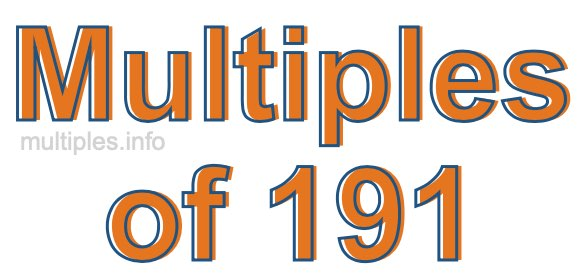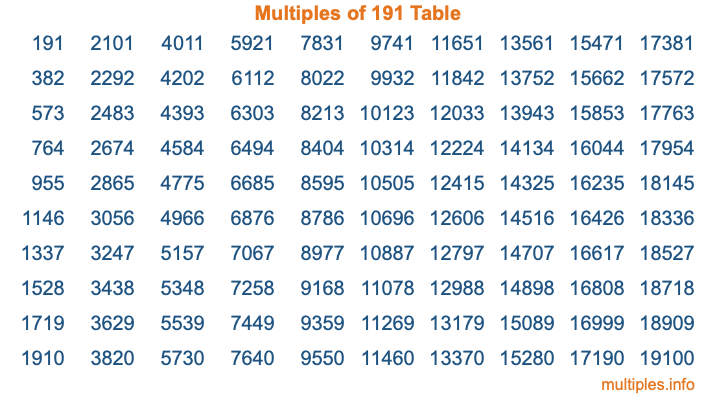Multiples of 191Welcome to the Multiples of 191 page. Here we will first teach you everything you will ever need to know about the multiples of 191, and then give you a study guide summary of everything we taught you to make sure you remember it all. Use this page to look up facts and learn information about the multiples of 191. This page will make you a multiples of one hundred ninety-one expert!

Definition of Multiples of 191
Multiples of 191 are all the numbers that when divided by 191 equal an integer. Each of the multiples of 191 are called a multiple. A multiple of 191 is created by multiplying 191 by an integer.

Therefore, to create a list of multiples of 191, you start with 1 multiplied by 191, then 2 multiplied by 191, then 3 multiplied by 191, and so on for as long as you want. Thus, the list of the first five multiples of 191 is 191, 382, 573, 764, and 955. To see a larger list of multiples of 191, see the printable image of Multiples of 191 further down on this page. We also have a category where you can choose any nth multiple of 191.

Multiples of 191 Checker
The Multiples of 191 Checker below checks to see if any number of your choice is a multiple of 191. In other words, it checks to see if there is any number (integer) that when multiplied by 191 will equal your number. To do that, we divide your number by 191. If the the quotient is an integer, then your number is a multiple of 191.

Is  a multiple of 191?

Least Common Multiple of 191 and ...
A Least Common Multiple (LCM) is the lowest multiple that two or more numbers have in common. This is also called the smallest common multiple or lowest common multiple and is useful to know when you are adding our subtracting fractions. Enter one or more numbers below (191 is already entered) to find the LCM.

Check out our LCM Calculator if you need more details about the Least Common Multiple or if you need the LCM for different numbers for adding and subtraction fractions.

nth Multiple of 191
As we stated above, 191 is the first multiple of 191, 382 is the second multiple of 191, 573 is the third multiple of 191, and so on. Enter a number below to find the nth multiple of 191.

th multiple of 191

Multiples of 191 vs Factors of 191
191 is a multiple of 191 and a factor of 191, but that is where the similarities end. All postive multiples of 191 are 191 or greater than 191. All positive factors of 191 are 191 or less than 191.

Below is the beginning list of multiples of 191 and the factors of 191 so you can compare:

Multiples of 191: 191, 382, 573, 764, 955, etc.

Factors of 191: 1, 191

As you can see, the multiples of 191 are all the numbers that you can divide by 191 to get a whole number. The factors of 191, on the other hand, are all the whole numbers that you can multiply by another whole number to get 191.

It's also interesting to note that if a number (x) is a factor of 191, then 191 will also be a multiple of that number (x).

Multiples of 191 vs Divisors of 191
The divisors of 191 are all the integers that 191 can be divided by evenly. Below is a list of the divisors of 191.

Divisors of 191: 1, 191

The interesting thing to note here is that if you take any multiple of 191 and divide it by a divisor of 191, you will see that the quotient is an integer.

Multiples of 191 Table
Below is an image of the first 100 multiples of 191 in a table. The table is in chronological order, column by column. The first column has the first ten multiples of 191, the second column has the next ten multiples of 191, and so on.The Multiples of 191 Table is also referred to as the 191 Times Table or Times Table of 191. You are welcome to print out our table for your studies.

Negative Multiples of 191
Although not often discussed or needed in math, it is worth mentioning that you can make a list of negative multiples of 191 by multiplying 191 by -1, then by -2, then by -3, and so on, to get the following list of negative multiples of 191:

-191, -382, -573, -764, -955, etc.

Multiples of 191 Summary
Below is a summary of important Multiples of 191 facts that we have discussed on this page. To retain the knowledge on this page, we recommend that you read through the summary and explain to yourself or a study partner why they hold true.

There are an infinite number of multiples of 191.

A multiple of 191 divided by 191 will equal a whole number.

191 divided by a factor of 191 equals a divisor of 191.

The nth multiple of 191 is n times 191.

The largest factor of 191 is equal to the first positive multiple of 191.

191 is a multiple of every factor of 191.

191 is a multiple of 191.

A multiple of 191 divided by a divisor of 191 equals an integer.

191 divided by a divisor of 191 equals a factor of 191.

Any integer times 191 will equal a multiple of 191.

Multiples of a Number
Here you can get the multiples of another number, all with the same attention to detail as we did for multiples of 191 on this page.

Multiples of
Multiples of 192
Did you find our page about multiples of one hundred ninety-one educational? Do you want more knowledge? Check out the multiples of the next number on our list!